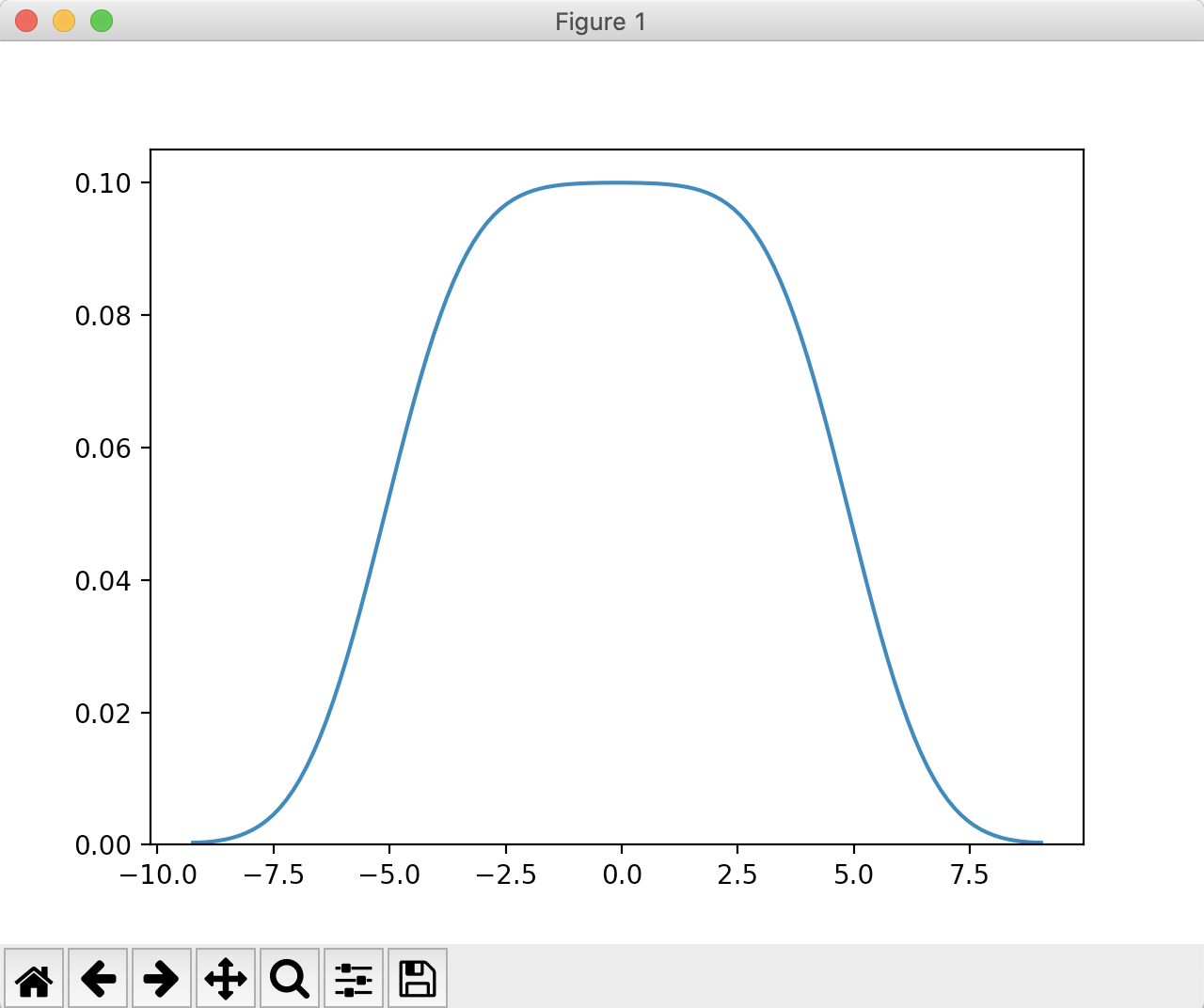# Drawing A Kernel Density Estimate(KDE) Plot Using Seaborn

## Overview:

• In statistics, Kernel Density Estimation is a non-parametric technique that calculates and plots the probability distribution(the probability density) of a continuous random variable. i.e., The calculation does not assume the underlying data to be following the assumptions of a normal distribution or any distribution.
• In simple terms, Kernel Density Estimate is like a smoothened counterpart of a histogram without the line of histogram intervals and their end-points.
• Such a smoothened curve for the probability density of a given data is obtained by drawing individual estimates for the data points and summing them up to produce the final contour.
• The bandwidth 'h' used in the estimation plays a role in the level of smoothness of the estimated curve. The lower the 'h' - more closer to the data and more spiky the curve is. When the value of 'h' is higher the resultant curve is over smoothend.

## KDE Plot in seaborn:

• Probablity Density Estimates can be drawn using any one of the kernel functions - as passed to the parameter "kernel" of the seaborn.kdeplot() function. By default, a Guassian kernel as denoted by the value "gau" is used. The kernels supported and the corresponding values are given here.
 Name of the kernel function Value of the parameter Guassian kernel "gau" Cosine "cos" Biweight "bi" Triweight "trw" Triangular "tri" Epanechnikov "epa"
• In seaborn the bandwidth of the KDE plot is controlled through the function parameter "bw".

Example:

 # Example Python program that draws a KDE plot # using a normal kernel import numpy as np import seaborn as sbn import matplotlib.pyplot as plt # Generate data points data = np.arange(-5, 5, 0.2); # Use gaussian kernel to plot the Kernel Density Estimation sbn.kdeplot(data); plt.show();

Output: